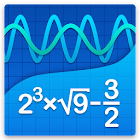# 科学图形计算器 Mathlab

78K 9

9 用户

## 截屏

* 算术表达式 +, - ，*，/，÷
* 平方根，立方和多次方根 （保持‘√’密钥）
* 指数，对数 （ln，log）
* 三角函数sin π/2，cos 30°，...
* 双曲线函数：正弦，余弦，正切，...（按“e”键切换）
* 反函数（按直接功能键）
* 复数，所有功能都支持复数
* 导数 sin x' = cos x，... （按 x^n 键）
* 科学记数法（在菜单中启用）
* 百分比模式
* 保存/载入历史

* 多种功能绘图
* 隐函数的第二度（椭圆 2x^2+3y^2=1，等等）
* 极性图 （r=cos2θ）
* 参数函数，输入新线 （x=cos t，y=sin t）
* 功能根和交叉点的图表，请点选的传说开启和关闭（左上角），使用菜单显示為一个列表
* 图交叉口 （x^2=x+1）
* 跟踪函数值和斜坡
* 滚动和缩放图表
* 捏放大
* 横向全屏图
* 函数表
* 保存為图像图形
* 表保存為 CSV

* 简单和复杂的分数 1/2+1/3=5/6
* 混合数字时，使用空格输入值 3 1/2

* 线性方程 x+1=2 -> x=1
* 二次方程 x^2-1= 0 -> x=-1,1
* 较高多项式近似根
* 系统线性方程组，每行写一个方程式，x1+x2=1，x1-x2=2
* 多项式长除法
* 多项式展开，多项式展开，因式分解

* 矩阵和向量运算
* 点击点积 （按住*），多种功能的图形
* 行列式，逆，规范，移调，跟踪库

* 用户定义的常量和函数（PRO）
* 保存/加载表达式

Tags: mathlab graphics calculato

78.019 来自评论

"非常棒"

9